# Example No. D5(a) Multifamily Dwelling Served at 208Y/120 Volts, Three Phase

### Example No D5(a) Multifamily Dwelling Served at 208Y 120 Volts, Three Phase

All conditions and calculations are the same as for the multifamily dwelling of Example No. D4(a) served at 120/240 volts, single phase except service to each dwelling unit would be two-phase legs and neutral.

Minimum Number of Branch Circuits Required for Each Dwelling Unit (See 220.11)

Range circuit: 8000 volt-amperes ÷ 208 volts = 38 amperes or a circuit of two 8 AWG conductors and one 10 AWG conductor as per 210.19(A)(3).

Minimum Size Feeder Required for Each Dwelling Unit (See 215.2)

 For 120/208-volt, 3-wire system (without ranges)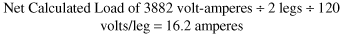For 120-208-volt, 3-wire system (with ranges) Net calculated load (range) of 8000 volt-amperes ÷ 208 volts = 38.5 amperes Total load (range plus lighting) = 38.5 VA + 16.2 A = 54.7 amperes Feeder neutral: (range) of 8000 VA x 70% = 5600 volt-amperes ÷ 208 volts = 26.9 amperes Total load (range plus lighting) = 26.9 A + 16.2 A = 43.1 A

Minimum Size Feeders Required from Service Equipment to Meter Bank (for 20 Dwelling Units10 with Ranges)

 For 208Y/120-volt, 3-phase, 4-wire system: Ranges: maximum number between any two phase legs = 4.2 x 4 = 8. Table 220.55 demand = 23,000 volt-amperes Per phase demand: 23,000 volt-amperes ÷ 2 = 11,500 volt-amperes Equivalent 3-phase load = 34,500 volt-amperes Net calculated load (total): 40,590 volt-amperes + 34,500 volt-amperes = 75,090 volt-amperes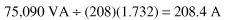Feeder Neutral Size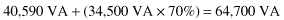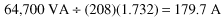Minimum Size Main Feeder (Less House Load) (for 40 Dwelling Units20 with Ranges)

 For 208Y/120-volt, 3-phase, 4-wire system: Ranges: maximum number between any two phase legs = 7 2 x 7 = 14. Table 220.55 demand = 29,000 volt-amperes Per phase demand: 29,000 VA ÷ 2 = 14,500 volt-amperes Equivalent 3-phase load = 43,500 volt-amperes Net Calculated Load (total): 69,150 volt-amperes + 43,500 volt-amperes = 112,650 volt-amperesMain Feeder Neutral Size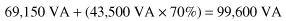99,600 volt-amperes ÷ (208)(1.732) = 276.5 amperes

Further Demand Factor (See 220.61)

 200 amperes at 100% 200.0 A 276.5 - 200 A = 76.5 amperes at 70% 53.6 A Net computed load neutral 253.6 A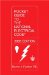Pocket Guide to the National Electrical Code(R), 2005 Edition (8th Edition)
ISBN: 0131480014
EAN: 2147483647
Year: 2004
Pages: 120